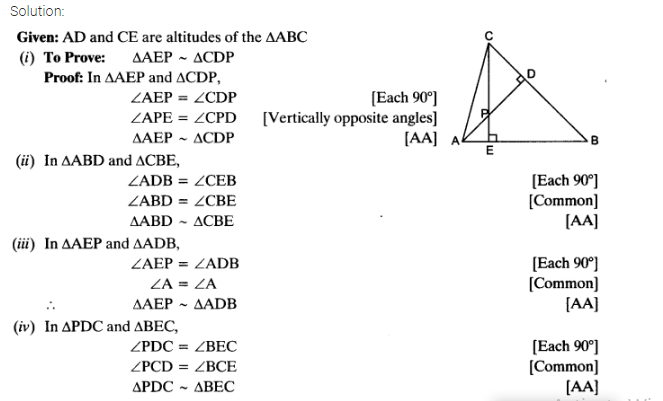• +91 9971497814
• info@interviewmaterial.com

# Triangles EX-6.3 Interview Questions Answers

### Related Subjects

Question 1 :
State which pairs of triangles in the given figures are similar. Write the similarity criterion used by you for answering the question and also write the pairs of similar triangles in the symbolic form :

Answer 1 :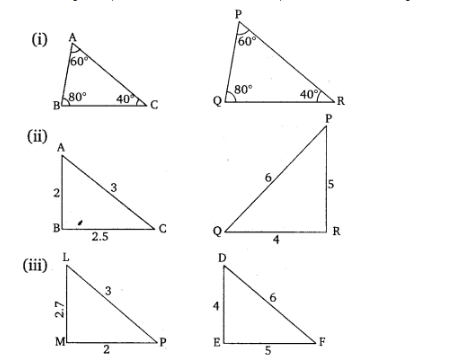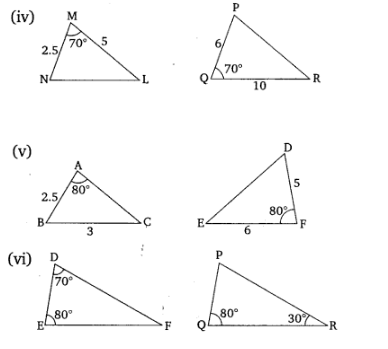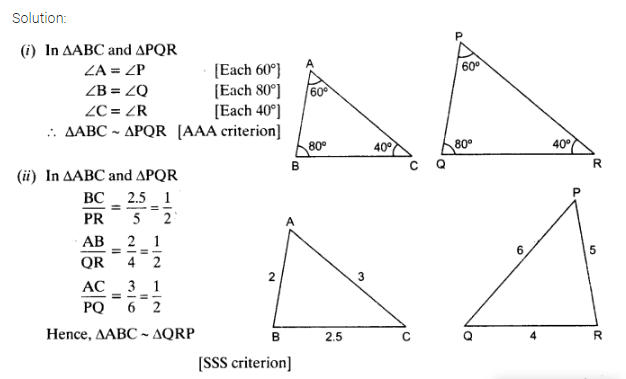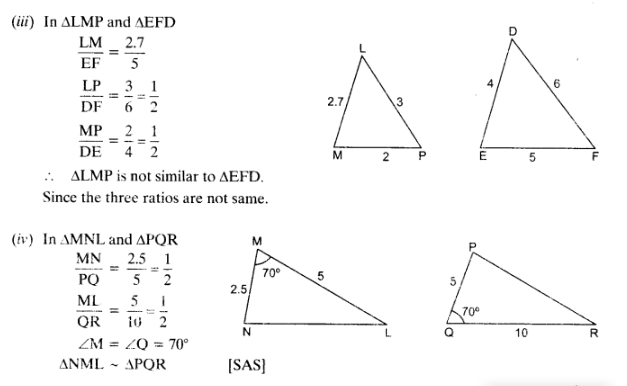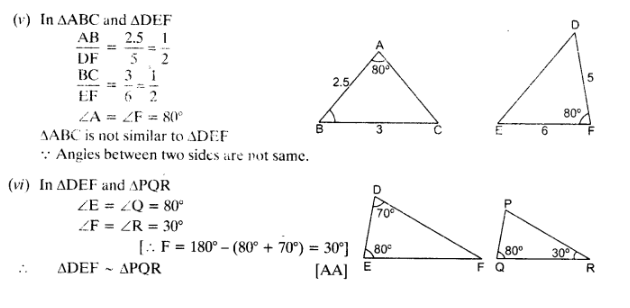Question 2 : In the given figure, ∆ODC ~ ∆OBA, ∠BOC = 125° and ∠CDO = 70°. Find ∠DOC, ∠DCO and ∠OAB.

Answer 2 :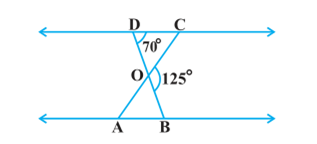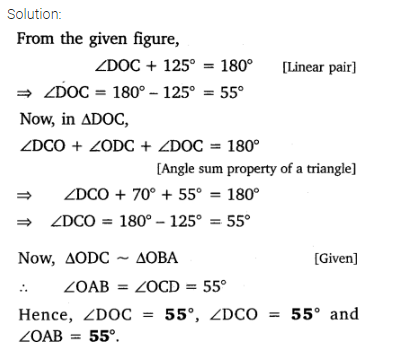Question 3 : Diagonals AC and BD of a trapezium ABCD with AB || DC intersect each other at the point O. Using a similarity criterion for two triangles, show that AO/OC = OB/OD

Answer 3 :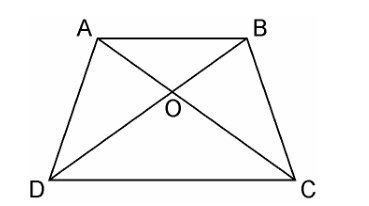In ΔDOC and ΔBOA,

AB || CD, thus alternate interior angles willbe equal,

∴∠CDO = ABO

Similarly,

DCO = BAO

Also, for the two triangles ΔDOC and ΔBOA,vertically opposite angles will be equal;

∴∠DOC = BOA

Hence, by AAA similarity criterion,

ΔDOC ~ ΔBOA

Thus, the corresponding sides areproportional.

DO/BO = OC/OA

OA/OC = OB/OD

Hence, proved.

Question 4 :

In the fig.6.36,QR/QS = QT/PR and 1 = 2. Show that ΔPQS ~ ΔTQR.

Answer 4 :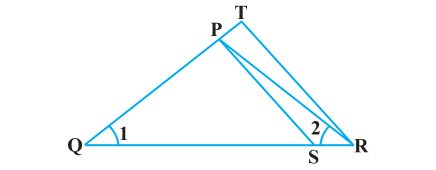Solution:

In ΔPQR,

PQR = PRQ

PQ = PR ………………………(i)

Given,

QR/QS = QT/PRUsing equation (i), we get

QR/QS = QT/QP……………….(ii)

In ΔPQS and ΔTQR, by equation (ii),

QR/QS = QT/QP

Q = Q

ΔPQS ~ ΔTQR [By SAS similarity criterion]

Question 5 : S and T are point on sides PR and QR of ΔPQRsuch that P= RTS. Show that ΔRPQ ~ ΔRTS.

Answer 5 :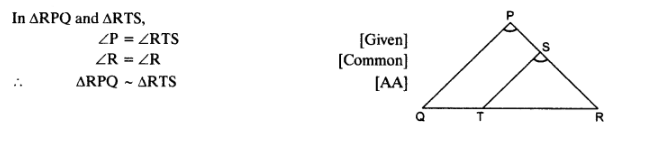Question 6 :

In the figure, ifΔABE ΔACD, show thatΔADE ~ ΔABC.

Answer 6 :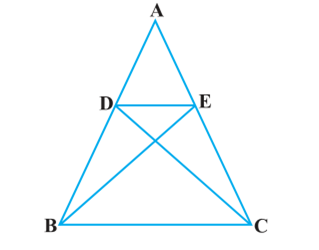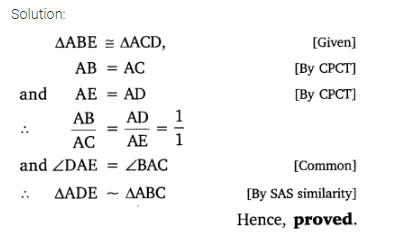Question 7 :

In the figure,altitudes AD and CE of ΔABC intersect each other at the point P. Show that:

Answer 7 :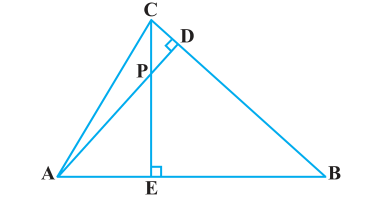(i) ΔAEP ~ ΔCDP
(ii) ΔABD ~ ΔCBE